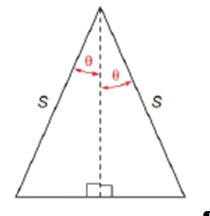Chapter 11.2, Problem 41E### Elementary Geometry for College St...

6th Edition
Daniel C. Alexander + 1 other
ISBN: 9781285195698

#### Solutions

Chapter
Section### Elementary Geometry for College St...

6th Edition
Daniel C. Alexander + 1 other
ISBN: 9781285195698
Textbook Problem
1 views

# Use the drawing provided to show that the area of the isosceles triangle is A = s 2 sin θ cos θTo determine

To find:

To show that the area of the isosceles triangle is A=s2sinθcosθ by using the following figure,Explanation

Consider the following figure,

Let us put the name for the isosceles triangle ia ABC and altitude is AD¯

We know that BD=DC=base2

Assume that BD=DC=xunits

General formula for cosine ratio and sin ratio is given below,

From the above figure shows that the s is the value of hypotenuse since it is opposite to right angle.

Then DC is the length of the leg opposite to θ.

Therefore,

### Still sussing out bartleby?

Check out a sample textbook solution.

See a sample solution

#### The Solution to Your Study Problems

Bartleby provides explanations to thousands of textbook problems written by our experts, many with advanced degrees!

Get Started

#### Convert the expressions in Exercises 6584 to power form. xy23

Finite Mathematics and Applied Calculus (MindTap Course List)

#### y-intercept 6, parallel to the line 2x + 3y + 4 = 0

Single Variable Calculus: Early Transcendentals, Volume I

#### In Exercises 69-74, rationalize the numerator. 69. 2x3

Applied Calculus for the Managerial, Life, and Social Sciences: A Brief Approach

#### Evaluate the integral. 01(18v3+16v7)dv

Single Variable Calculus: Early Transcendentals

#### Evaluate 734(916+212) .

Mathematics For Machine Technology